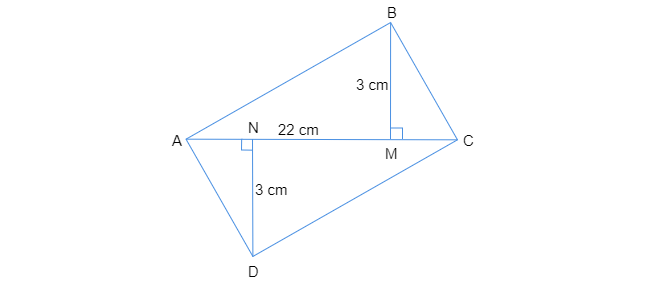DN = 3 cm ">

# Find the area of the quadrilateral ABCD. Here, AC = 22 cm, BM = 3 cm,DN = 3 cm

Given: $AC = 22\ cm,\ BM = 3\ cm,\ DN = 3\ cm$

To do: To find the area of the quadrilateral ABCD.

Solution:

Area of the $∆ ABC = \frac{1}{2}\times base\times height$

$=\frac{1}{2}\times AC\times BM$

$=\frac{1}{2}\times 22\ cm\times 3\ cm$

$=33\ cm^2$

Area of the $∆ ADC=\frac{1}{2}\times base\times height$

$=\frac{1}{2}\times AC\times DN$

$= 1/2\times 22\times 3$

$= 33\ cm^2$

Area of the quadrilateral $ABCD=$Area of the $∆ ABC +$Area of the $∆ ADC$

$=33\ cm^2 + 33\ cm^2$

$=66\ cm^2$

Updated on: 10-Oct-2022

23 Views